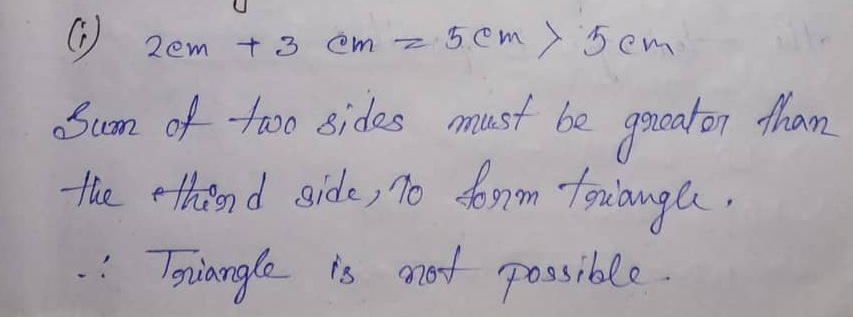# ML Aggarwal CBSE Solutions Class 7 Math Tenth Chapter Triangle and its Properties Exercise 10.4

### ML Aggarwal CBSE Solutions Class 7 Math 10th Chapter Triangle and its Properties Exercise 10.4

(1) Is it possible to have a triangle with the following sides?

(i) 2 cm, 3 cm, 5 cm

(ii) 2.5 cm, 4.5 cm, 8 cm

(iii) 10.2 cm, 5.8 cm, 4.5 cm

(iv) 3.4 cm, 4.7 cm, 6.2 cm.

Solution:(2) If the lengths of two sides of a triangle are 7 cm and 10 cm, then what can be the length of the third side?

(3) We know that in a triangle, the sum of lengths of any two sides is greater than the length of the third side. Is the sum of any angles of a triangle also greater than the third angle? If no, draw a rough sketch to show such a case.

Solution:Updated: November 7, 2019 — 1:16 pm